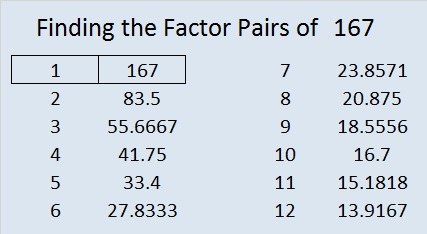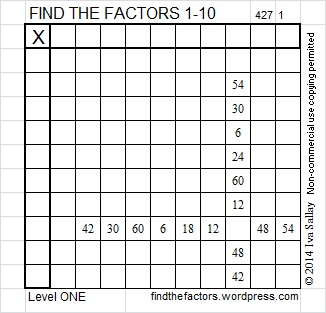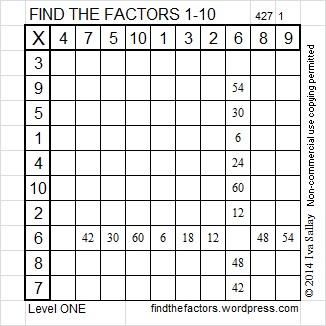# 167 and Level 1

• 167 is a prime number.
• Prime factorization: 167 is prime.
• The exponent of prime number 167 is 1. Adding 1 to that exponent we get (1 + 1) = 2. Therefore 167 has exactly 2 factors.
• Factors of 167: 1, 167
• Factor pairs: 167 = 1 x 167
• 167 has no square factors that allow its square root to be simplified. √167 ≈ 12.9228How do we know that 167 is a prime number? If 167 were not a prime number, then it would be divisible by at least one prime number less than or equal to √167 ≈ 12.9. Since 167 cannot be divided evenly by 2, 3, 5, 7, or 11, we know that 167 is a prime number.Excel file of puzzles and previous week’s factor solutions: 10 Factors 2014-07-07This site uses Akismet to reduce spam. Learn how your comment data is processed.